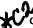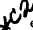Section   XVII. B R A K E    C O O L A N T    O I L    P U M P  R E B U I LD
Custom Search*C2 *C2 *C2 C H A P   5,   SEC  X V II B R A K E    C O O L A N T    O I L    P U M P    R E B U I LD PAR   147-151 Section   XVII. B R A K E     C O O L A N T     O I L     P U M P — R E B U I LD 1 4 7 .    D E S C R I P T I ON R e f e r    t o    p a r .    2 4    f o r    d e s c r i p t i o n    o f    t h e brake   coolant   oil   pump. 148.    DISASSEMBLY    (fig.    384,    fold-out    13) .   U s i n g     a     9 / 1 6 - i n c h     w r e n c h ,     r e m o ve three   cap   screws   60   with   three   lock   washers 59   and   three   self-locking   bolts   53   securing   the brake   coolant   pump   assembly   20   to   the   brake coolant   pump   manifold   56.   Remove   the   pump assembly   and   gasket   54. .   Remove   two   sets   of   brake   coolant   oil check   valve   components   (64   through   68). .   U s i n g    l / 2 - i n c h    w r e n c h ,    r e m o v e    s e l f- locking   bolt   51   securing   drive   gear   shroud   48 and   shroud   plate   50   to   the   pump.   Remove   the shroud   and   plate.   Straighten   and   remove   lock pin   42   from   gear   49.   Remove   the   gear. .   U s i n g    a    l / 2 - i n c h    w r e n c h ,    r e m o v e    14 self-locking   bolts   27   and   52,   securing   the   two cover   assemblies   28   and   44   to   the   coolant   oil pump   body   32.   Remove   the   cover   assemblies. .  Do   not   remove   the   needle   bearing   as- semblies   29   and   45   or   dowel   pins   31   and   46 from   covers   30   and   47   unless   replacement   is n e c e s s a r y .    I f    n e c e s s a r y ,    p r e s s    o r    d r i v e    o ut the   bearings   or   pins. .   R e m o v e ,    a s    a    u n i t ,    i d l e r    g e a r    s h a f t 22,   pump   drive   shaft   40,   two   oil   pump   idler gears   21   and   26,   two   drive   gears   33   and   43, and   the   two   oil   pump   separator   plate   assem- blies   23   and   36   from   the   pump   body. .   R e m o v e    i d l e r    g e a r s    2 1    a n d    2 6    f r om their   shaft   22. .   Remove   oil   pump   drive   gears   33   and 43   and   keys   34   and   41   from   the   pump   drive shaft   40. .   R e m o v e    t w o    r e t a i n i n g    r i n g s    3 5    f r om oil  oil  pump  idler  22  and  drive  40  gear  shafts. j.   Remove   separator   plate   assemblies   23 and   36   from   shafts   22   and   40. 1 2 8 .   Do   not   remove   needle   bearings   25   and 37   from   separator   plates   24   and   38   unless   re- p l a c e m e n t    i s    n e c e s s a r y .    I f    n e c e s s a r y ,    p r e ss b e a r i n g s    f r o m    p l a t e s. .   Do   not   remove   retaining   rings   39   from oil   pump   idler   22   and   drive   40   gear   shafts   un- l e s s    r e p l a c e m e n t    i s    n e c e s s a r y. 1 4 9 .    C L E A N I NG Refer   to   par.   71   for   cleaning   recommen- dations. 1 5 0 .    I N S P E C T I O N    A N D    R E P A IR R e f e r    t o    p a r .    7 2    f o r    g e n e r a l    i n s p e c t i on and   repair   recommendations.   Repair   and   re- b u i l d    p o i n t s    o f    m e a s u r e m e n t    f o r    f i t s ,    c l e a r- ances   and   wear   limits   are   indicated   by   small, l o w e r    c a s e    l e t t e r s    i n    f i g .    3 8 4 ,    f o l d - o u t    1 3. Refer   to   par.   247   for   wear   limits   information. 1 5 1 .    A S S E M B L Y    ( f i g .    3 8 4 ,    f o l d - o u t    1 3) .   If   needle   bearings   25   and   37   were   re- moved   from   separator   plates   24   and   38,   install replacements 0.320-inch below the surface a d j a c e n t    t o    t h e    b e a r i n g    b o r e s .    P r e s s    o n    t h e numbered   side   of   the   bearing   cage.Integrated Publishing, Inc. - A (SDVOSB) Service Disabled Veteran Owned Small Business